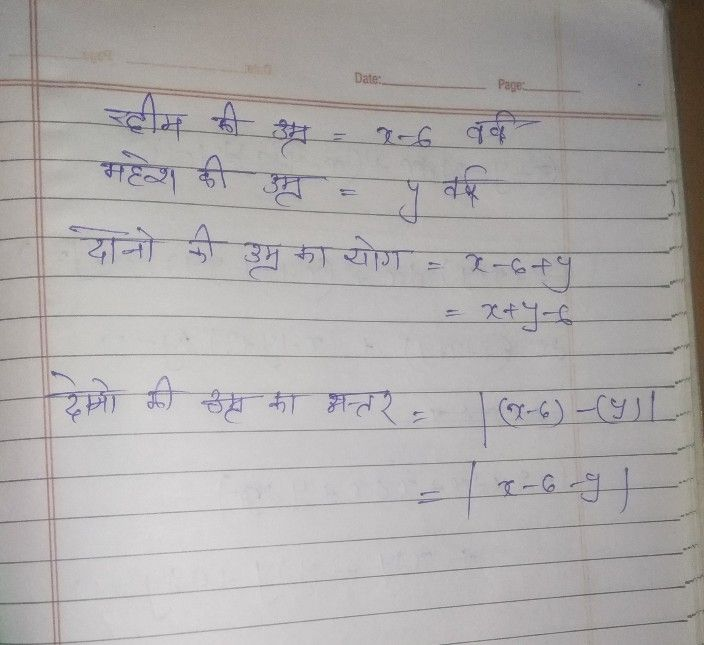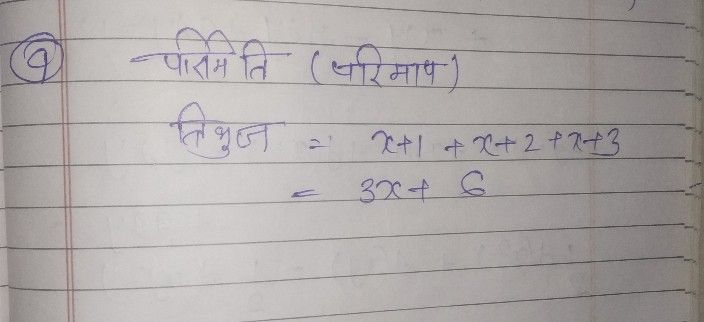Symbol
Problem$0+c-0a+30+7c$ $\left($ (d) $\right)$ $8x^{2}+5xy+3y^{2}+3x^{2}+2xy-6y^{2}$ $4.$ $3$ $x+1,x+2\bar{SG}$ $x+3$ $5$ $5$ $3$ $\bar{\sqrt{7} } 1$ $x-7$ $6.$ $x-6$ $y$
Algebra
SolutionQanda teacher - priyanka